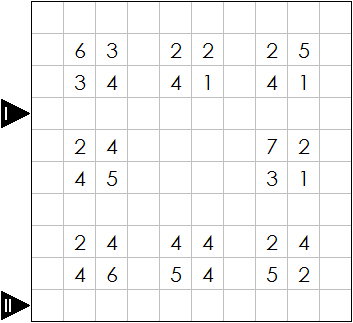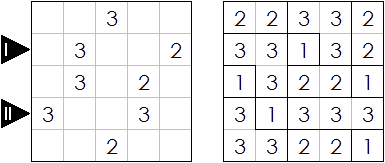# Fillomino

Logic Level 5(Right click on the image and click "View image" to see a larger, clearer version.)

Fillomino is a logic puzzle devised by nikoli. You are given a grid, partially filled with numbers; your task is to divide the grid into regions such that:

• Each number indicates the area its region is contained in,
• No two regions with equal area are orthogonally adjacent (sharing a side; touching by a corner is okay).

Note that a region may be clued by multiple numbers, or even no number at all.

A sample puzzle (left) with its solution (right) can be seen below.Your task is to solve the Fillomino puzzle above (at the very top).

After solving it and filling each cell with the area of its region, submit the digits in the first marked row (row 4), followed by the digits in the second marked row (row 10). (For double-digit cells, put both digits.) For the example above, the answer is $3313231333$.

×